Two-order Runge-Kutta Method using Mathematica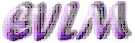Аnotation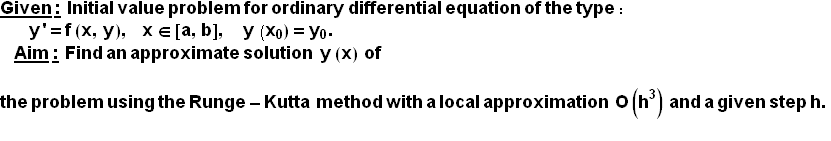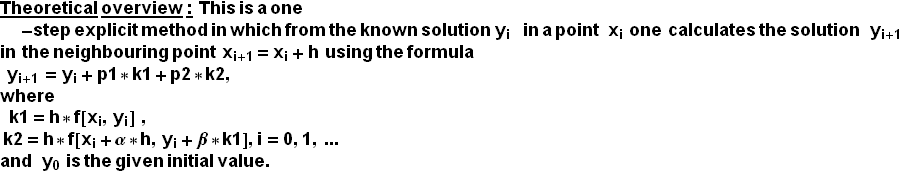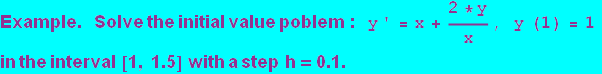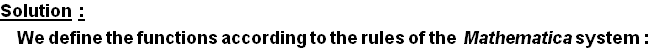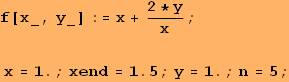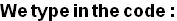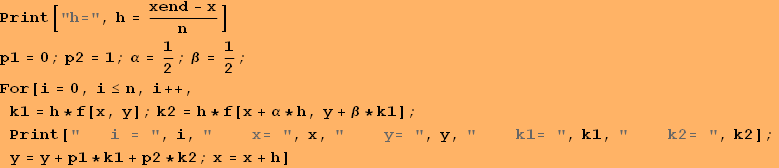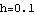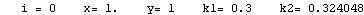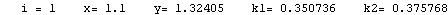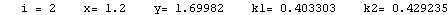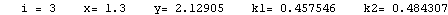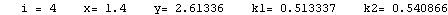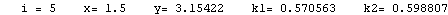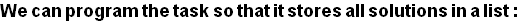1 1 1.1 1.32405 1.2 1.69982 1.3 2.12905 1.4 2.61336 1.5 3.15422

Conclusion:   The solution derived using the Runge-Kutta method O(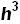) is in the form of a table of the variable function y(x), shown in the second column of the table above.  Since the  local apriori error of the method is O() (or global error is  O(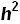) ) and here h=0.1, then these solutions must be rounded to three symbols after the decimal point.

Graphic of the solution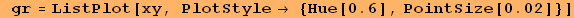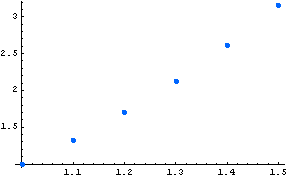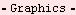Created by Wolfram Mathematica 6.0  (22 September 2008)